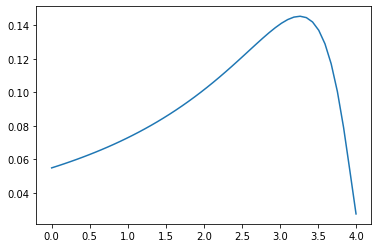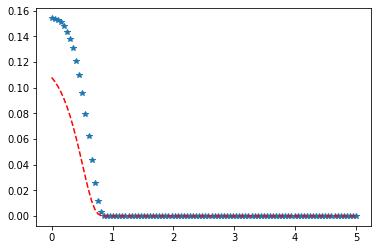# Python – Left-skewed Levy Distribution in Statistics

scipy.stats.levy_l() is a left-skewed Levy continuous random variable. It is inherited from the of generic methods as an instance of the rv_continuous class. It completes the methods with details specific for this particular distribution.

Parameters :

q : lower and upper tail probability
x : quantiles
loc : [optional]location parameter. Default = 0
scale : [optional]scale parameter. Default = 1
size : [tuple of ints, optional] shape or random variates.
moments : [optional] composed of letters [‘mvsk’]; ‘m’ = mean, ‘v’ = variance, ‘s’ = Fisher’s skew and ‘k’ = Fisher’s kurtosis. (default = ‘mv’).

Results : left-skewed Levy continuous random variable

Code #1 : Creating left-skewed Levy continuous random variable

 `# importing library ` ` `  `from` `scipy.stats ``import` `levy_l   ` `   `  `numargs ``=` `levy_l.numargs  ` `a, b ``=` `4.32``, ``3.18` `rv ``=` `levy_l(a, b)  ` `   `  `print` `(``"RV : \n"``, rv)   `

Output :

```RV :
scipy.stats._distn_infrastructure.rv_frozen object at 0x000002A9D6707508
```

Code #2 : left-skewed Levy continuous variates and probability distribution

 `import` `numpy as np  ` `quantile ``=` `np.arange (``0.03``, ``2``, ``0.21``)  ` ` `  `# Random Variates  ` `R ``=` `levy_l.rvs(a, b)  ` `print` `(``"Random Variates : \n"``, R)  ` ` `  `# PDF  ` `R ``=` `levy_l.pdf(a, b, quantile)  ` `print` `(``"\nProbability Distribution : \n"``, R)  `

Output :

```Random Variates :
1.1073459342251062

Probability Distribution :
[0. 0. 0. 0. 0. 0. 0. 0. 0. 0.]

```

Code #3 : Graphical Representation.

 `import` `numpy as np  ` `import` `matplotlib.pyplot as plt  ` `    `  `distribution ``=` `np.linspace(``0``, np.maximum(rv.dist.b, ``4``))  ` `print``(``"Distribution : \n"``, distribution)  ` `    `  `plot ``=` `plt.plot(distribution, rv.pdf(distribution))   `

Output :

```Distribution :
[0.         0.08163265 0.16326531 0.24489796 0.32653061 0.40816327
0.48979592 0.57142857 0.65306122 0.73469388 0.81632653 0.89795918
0.97959184 1.06122449 1.14285714 1.2244898  1.30612245 1.3877551
1.46938776 1.55102041 1.63265306 1.71428571 1.79591837 1.87755102
1.95918367 2.04081633 2.12244898 2.20408163 2.28571429 2.36734694
2.44897959 2.53061224 2.6122449  2.69387755 2.7755102  2.85714286
2.93877551 3.02040816 3.10204082 3.18367347 3.26530612 3.34693878
3.42857143 3.51020408 3.59183673 3.67346939 3.75510204 3.83673469
3.91836735 4.        ]
```Code #4 : Varying Positional Arguments

 `import` `matplotlib.pyplot as plt  ` `import` `numpy as np  ` `    `  `x ``=` `np.linspace(``0``, ``5``, ``100``)  ` `    `  `# Varying positional arguments  ` `y1 ``=` `levy_l .pdf(x, ``1``, ``3``)  ` `y2 ``=` `levy_l .pdf(x, ``1``, ``4``)  ` `plt.plot(x, y1, ``"*"``, x, y2, ``"r--"``)  `

Output :My Personal Notes arrow_drop_upCheck out this Author's contributed articles.

If you like GeeksforGeeks and would like to contribute, you can also write an article using contribute.geeksforgeeks.org or mail your article to contribute@geeksforgeeks.org. See your article appearing on the GeeksforGeeks main page and help other Geeks.

Please Improve this article if you find anything incorrect by clicking on the "Improve Article" button below.

Article Tags :

Be the First to upvote.

Please write to us at contribute@geeksforgeeks.org to report any issue with the above content.